# User Guide: 3 Plotting transformed data

## Introduction

Package ggspectra extends ggplot2 with stats, geoms, scales and annotations suitable for light spectra. It also defines ggplot() and autoplot() methods specialized for the classes defined in package photobiology for storing different types of spectral data. The autoplot() methods are described separately in vignette User Guide: 2 Autoplot Methods and the ggplot() methods, statistics, and scales in User Guide: 1 Grammar of Graphics.

The new elements can be freely combined with methods and functions defined in packages ‘ggplot2’, scales, ggrepel, cowplot and other extensions to ‘ggplot2’.

This third part of the User Guide describes how to combine manipulation of spectral data with plotting. This streamlined coding is made possible by the enhancement implemented in ‘ggspectra’ (>= 0.3.5). In addition, some of the examples make use of methods available only in ‘photobiology’ (>= 0.9.30).

In ‘ggspectra’ (>= 0.3.5) the data member of gg (ggplot) objects remains as an object of the classes for spectral data defined in ‘photobiology’ instead of being converted into a plain data.frame. This makes it possible data manipulations in layers to be done with methods specific to spectral data.

## Set up

library(ggplot2)
library(dplyr)
library(rlang)
library(photobiology)
library(photobiologyWavebands)
library(ggspectra)

good_label_repel <- packageVersion('ggrepel') != "0.8.0" #||
#  packageVersion('ggplot2') >= "3.1.0"

Create a collection of two source_spct objects.

two_suns.mspct <- source_mspct(list(sun1 = sun.spct, sun2 = sun.spct / 2))

We bind the two spectra in the collection into a single spectral object. This object includes an indexing factor, by default names spct.idx. We use this new object to later on demonstrate grouping in ggplots.

two_suns.spct <- rbindspct(two_suns.mspct)

We change the default theme.

theme_set(theme_bw())

## Visualizing the effect of methods

As from version 0.3.5 the class of the spectral object stored by ggplot() is no longer stripped, data transformations in layers can use methods from package ‘photobiology’ that expect spectral objects as input. A simple example of spectral data smoothing follows.

ggplot() +
geom_line(data = sun.spct, mapping = aes(w.length, s.e.irrad)) +
geom_line(data = sun.spct %>% smooth_spct(method = "supsmu"),
colour = "red", size = 1.2)

Can be now simplified because . refers to a data member of class source_spct while the automatic mapping remains valid because smooth_spct() returns a new source_spct object with the same column names as sun.spct.

ggplot(sun.spct) +
geom_line() +
geom_line(data = . %>% smooth_spct(method = "supsmu"),
colour = "red", size = 1.2)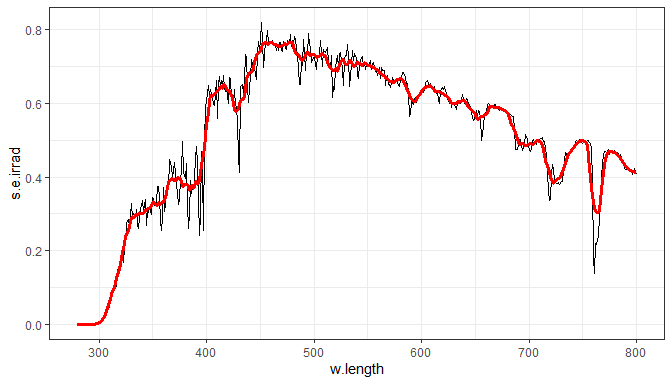photon_as_default()
ggplot(sun.spct) +
geom_line() +
geom_line(data = . %>% smooth_spct(method = "supsmu"),
colour = "red", size = 1.2)
unset_radiation_unit_default()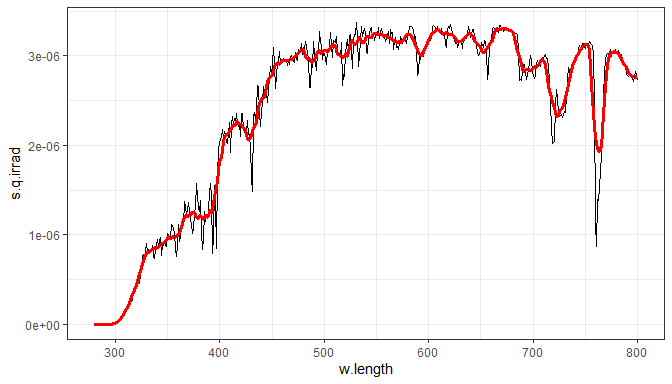Obviously, the default data does not need to be plotted, so this provides a roundabout way of applying methods,

ggplot(sun.spct) +
geom_line(data = . %>% smooth_spct(method = "supsmu"),
colour = "red", size = 1.2)

which is equivalent to doing the transformation ahead of plotting, which might be preferable.

sun.spct %>%
smooth_spct(method = "supsmu") %>%
ggplot() +
geom_line(colour = "red", size = 1.2)

However, when using different transformations in different layers we need to apply them at each layer.

ggplot(sun.spct) +
geom_line() +
geom_line(data = . %>% smooth_spct(method = "custom"),
colour = "blue", size = 1) +
geom_line(data = . %>% smooth_spct(method = "lowess"),
colour = "green", size = 1) +
geom_line(data = . %>% smooth_spct(method = "supsmu"),
colour = "red", size = 1)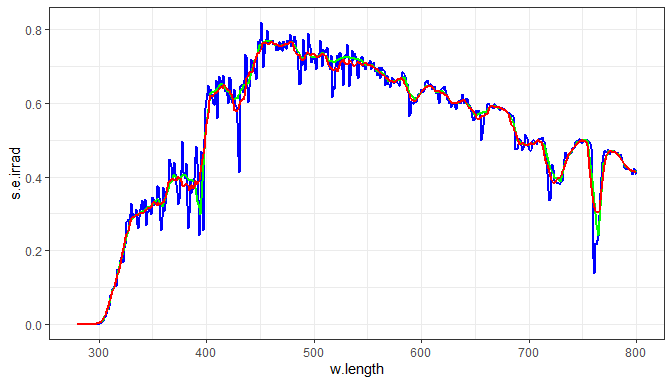Of course, this approach works both with geoms and stats, but one should remember that these layer functions do not “see” the original data object, but instead a new data frame containing the mapped variables in columns named according to aesthetics. The next example demostrates this and illustrates that smoothing displaces the wavelength of maximum spectral irradiance.

ggplot(sun.spct) +
geom_line() +
stat_peaks(size = 3, span = NULL) +
stat_peaks(geom = "vline", linetype = "dotted", span = NULL) +
geom_line(data = . %>% smooth_spct(method = "supsmu"),
colour = "red", size = 1.2) +
stat_peaks(data = . %>% smooth_spct(method = "supsmu"),
size = 3, span = NULL) +
stat_peaks(data = . %>% smooth_spct(method = "supsmu"),
geom = "vline", linetype = "dotted", span = NULL)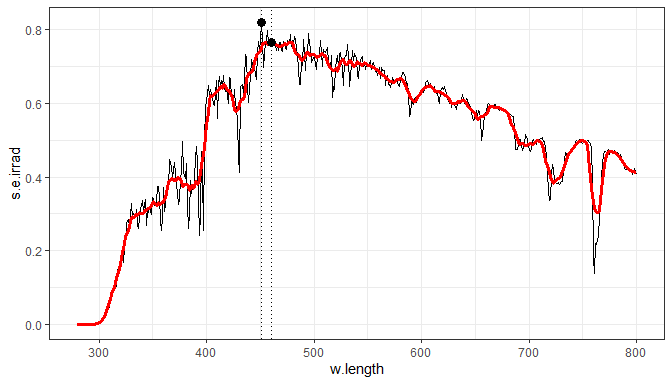We can easily highlight a wavelength region.

ggplot(sun.spct) +
geom_line() +
geom_line(data = . %>% trim_wl(range = PAR()), colour = "red")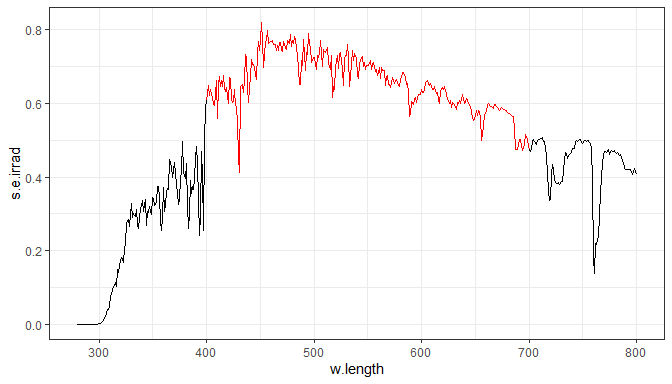ggplot(sun.spct) +
geom_line() +
geom_point(data = . %>% trim_wl(range = VIS()) %>% tag(),
mapping = aes(color = wl.color),
shape = "circle", size = 1.3) +
scale_color_identity()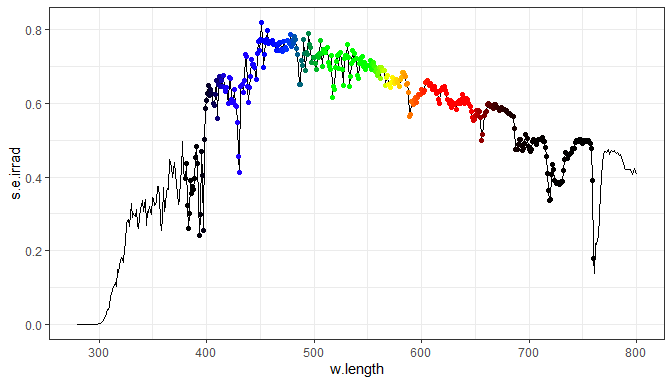In the plot above, spectral irradiance is taken into account when computing the colors, while below, only the wavelength at the centre of each waveband is used.

ggplot(sun.spct) +
geom_area(data = . %>% trim_wl(range = VIS()) %>% tag(w.band = VIS_bands()),
mapping = aes(fill = wb.color)) +
geom_line() +
scale_fill_identity()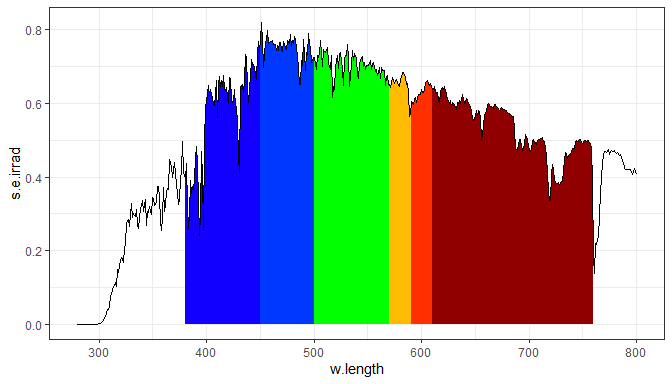Some of the methods from ‘photobiology’ are also defined for data.frame and can be used as summary functions with data that are not radiation spectra, such as any data seen by layer functions including stat_summary().